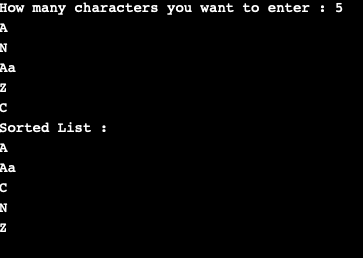# Java Program to Sort ArrayList of Custom Objects By Property

• Last Updated : 17 Nov, 2020

Here we are going to look at the approach of sorting an ArrayList of custom objects by using a property.

Approach:

1. Create a getter function which returns the value stored in the class variable.

2. Create a list and use sort() function which takes the values of the list as arguments and compares them with compareTo() method.

3. This function will then return a positive number if the first argument’s property is greater than the second’s, negative if it is less and zero if they are equal.

Implementation

## Java

 `// Java Program to Sort ArrayList of Custom Objects By``// Property`` ` `import` `java.util.*;`` ` `public` `class` `Main {``    ``private` `String value;`` ` `    ``public` `Main(String val) { ``this``.value = val; }`` ` `    ``// Defining a getter method``    ``public` `String getValue() { ``return` `this``.value; }`` ` `    ``// list of Main objects``    ``static` `ArrayList
list = ``new` `ArrayList<>();`` ` `    ``public` `static` `void` `sortList(``int` `length)``    ``{``        ``// Sorting the list using lambda function``        ``list.sort(``            ``(a, b) -> a.getValue().compareTo(b.getValue()));``        ``System.out.println(``"Sorted List : "``);`` ` `        ``// Printing the sorted List``        ``for` `(Main obj : list) {``            ``System.out.println(obj.getValue());``        ``}``    ``}`` ` `    ``public` `static` `void` `main(String[] args)``    ``{``        ``// take input``        ``Scanner sc = ``new` `Scanner(System.in);``        ``System.out.print(``            ``"How many characters you want to enter : "``);``        ``int` `l = sc.nextInt();`` ` `        ``// Taking value of list as input``        ``for` `(``int` `i = ``0``; i < l; i++) {``            ``list.add(``new` `Main(sc.next()));``        ``}`` ` `        ``sortList();``    ``}``}`

OutputMy Personal Notes arrow_drop_up